## Introduction

Galton stated the ancestral law as follows in 1897:

‘In the following memoir the truth will be verified in a particular instance, of a statistical law of heredity that appears to be universally applicable to bisexual descent...

The law to be verified... is that the two parents contribute between them on the average one-half, or (0.5) of the total heritage of the offspring; the four grandparents, one-quarter, or (0.5)2; the eight great-grandparents, one-eighth, or (0.5)3, and so on. Thus the sum of the ancestral contributions is expressed by the series {(0.5)+(0.5)2+(0.5)3, &c.}, which, being equal to 1, accounts for the whole heritage...

The law may be applied either to total values or to deviations, as will be gathered from the following equation. Let M be the mean value from which all deviations are reckoned, and let D1, D2, &c., be the means of all the deviations, including their signs, of the ancestors in the 1st, 2nd, &c., degrees respectively; then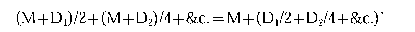Provine (1971) argues that this passage contains two distinct and mathematically inconsistent forms of the law, but his argument is not easy to understand. The real problem is to understand how Galton came to formulate a law of such striking generality, that underpinned the conflict between the Mendelians and the biometricians. The purpose of this paper is to clarify the rather confused arguments that he used to derive the ancestral law.

Galton was motivated to formulate the law by his work on regression published in 1885 (Galton, 1885). In this paper he showed that regression to the mean occurred when he plotted the height of offspring against mid-parental height (the average height of their two parents), the average offspring deviation from the mean (D0) being only two-thirds that of the mid-parent (D1):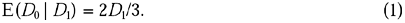He explained filial regression as resulting from reversion to ancestral values (‘The child inherits partly from his parents, partly from his ancestry’), together with the fact that ancestral values are likely to deviate less from the mean than the mid-parent does. This explanation led him to ask how much the child inherited, on average, from each of his ancestors, and hence to formulate the ancestral law. In the Introduction to Natural Inheritance (Galton, 1889) he framed the problem as follows:

A second problem regards the average share contributed to the personal features of the offspring by each ancestor severally. Though one half of every child may be said to be derived from either parent, yet he may receive a heritage from a distant progenitor that neither of his parents possessed as personal characteristics. Therefore the child does not on the average receive so much as one half of his personal qualities from each parent, but something less than a half. The question I have to solve, in a reasonable and not merely in a statistical way, is, how much less?

In this passage Galton distinguishes clearly between the phenotype (personal features) and the genotype (of which every child receives half from each parent). He believed, following Darwin (1868), that reversion, which gives rise to this distinction, occurs because only some of the hereditary elements (the patent or personal elements) are expressed, the rest being latent, that is to say, unexpressed but capable of transmission. In this context, it is natural to ask: What proportion of an individual's patent elements was patent in his (or her) parents? What proportion was latent in his parents but patent in one of the grandparents? and so on. The ancestral law asserts that the answers are one-half, one-quarter, and so on. The question is reasonable under the assumed model of heredity, although the answer given by the ancestral law may be wrong.

Some confusion has arisen from Karl Pearson's mistaken suggestion (Pearson, 1924, p. 84) that Galton had the ancestral law in mind in his first paper on heredity in 1865 (Galton, 1865):

‘And then follows Galton's first enunciation of the Law of Ancestral Heredity. He writes:

“The share that a man retains in the constitution of his remote descendants is inconceivably small. The father transmits, on an average, one-half of his nature, the grandfather one-fourth, the great-grandfather one-eighth; the share decreasing step by step, in a geometrical ratio, with great rapidity.”

Galton, 1865, p. 326

Galton is clearly on the right track, but the numbers he gives would only be correct if he used parental generation, grandparental generation, great-grandparental generation, instead of father, grandfather, great-grandfather. He has overlooked the mother, and overlooked the multiplicity of the ancestral individuals. The numbers as he gives them in a later publication are one-fourth for the parent, one-sixteenth for the grandparent and one-sixty-fourth for the great-grandparent, etc.... Thus in 1865 Galton had already in mind this law of ancestral heredity, although by an obvious oversight he gave the wrong proportions.’

Pearson's interpretation has been adopted by several authors (Swinburne, 1965, p. 22; Froggatt & Nevin, 1971a, pp. 5–6, and 1971b, p. 5; Cowan, 1977, p. 145), but the following is a more straightforward interpretation, which avoids the gratuitous assumption that Galton gave the wrong proportions by an oversight. If an individual inherits half his germ-plasm from his father (and half from his mother), and if the father inherits half of his germ-plasm from his father, then the individual must have inherited one-quarter of his germ-plasm from his paternal grandfather, and so on. The passage quoted is not a precursor of the law of ancestral heredity, but a restatement of the law of halving, known for a long time to breeders in terms of ‘blood fractions’. This law is a simple consequence of biparental inheritance, which was familiar to Galton in 1865. The general idea of reversion to ancestral characters underlying the ancestral law was known to him in 1865 under the name of the law of atavism, but he did not attempt to quantify it until he had undertaken the research into the statistical theory of heredity published in 1885.

Galton interpreted the ancestral law both as a representation of the separate contributions of each ancestor, on average, to the heritage of the offspring and as a prediction formula for predicting the value of a trait from ancestral values. He made several attempts to study inheritance over several generations to verify his law as a prediction formula; the most extensive data are on coat colour in basset hounds, obtained from pedigree records and published in 1897 (Galton, 1897). There are two coat colours in these hounds, tricolour (white, yellow and black) and nontricolour (white and yellow). Galton applied the total value form of the law to this all-or-nothing character by coding tricolour as 1 and nontricolour as 0: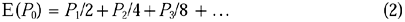where E(P0) is the predicted proportion of tricolour offspring in pedigrees with 2P1 tricolour parents, 4P2 tricolour grandparents, and so on; for example, the predicted proportion of tricolour offspring in a pedigree with two tricolour parents and three tricolour grandparents is 0.5+0.1875+0.1467=0.8342; 0.5 (P1/2) is the effect of the parents, 0.1875 (P2/4) is the effect of the grandparents, and 0.1467 is the estimated effect of the probable more remote ancestry, given three tricolour and one nontricolour grandparent (made up of 0.0408 for each tricolour and 0.0243 for each nontricolour grandparent). The good agreement between observed and predicted numbers shown in Table 1 encouraged Galton and his followers, particularly Pearson (1898), to believe in the validity of his law.

Before considering Galton's derivation of the ancestral law in detail, it is helpful to consider how a geneticist today would derive the law, given the theory of inheritance outlined by Galton in Natural Inheritance (1889).

## Logical reconstruction of the law

Galton supposed that inheritance is mediated through particulate elements in the germ-plasm. In bisexual inheritance each parent transmits half of his or her elements to the offspring, thus maintaining the total number of elements in successive generations. Elements may be latent or patent, only the patent ones being expressed, but a latent element may become patent in a subsequent generation, thus accounting for the phenomenon of reversion in which an individual expresses a character present in a distant ancestor but not in more recent ancestors. The simplest model incorporating these components is that a proportion, p, of the elements is patent in each individual, that patent and latent elements are equally likely to be transmitted to the offspring, and that all elements have the same chance, p, of being patent regardless of their status in the parent and more remote ancestors. This is the model implicitly assumed in Natural Inheritance (Galton, 1889), although it differs from the model presented in Galton (1872, 1875).

Under this model, the chance that a patent element in a parent will be present and patent in a child is p/2 (because it has a chance 1/2 of being present and independently a chance p of being patent); the chance that a patent element in a grandparent will be present and patent in a grandchild is p/4 (because it has a chance 1/4 of being present and independently a chance p of being patent); and so on. If a character such as height is determined additively by a number of patent elements, these probabilities are also the respective correlations, so that: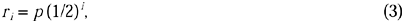where ri is the correlation between a child and a single ancestor i generations back. This is also the ancestral correlation under simple Mendelian models without epistasis if p is replaced by h2, the narrow heritability; thus Galton's model is formally equivalent to Mendelism, with latency replaced by dominance and environmental variability.

Interpreting the ancestral law as a prediction equation, the problem is to determine the partial regression coefficients, βi, in the multiple regression equation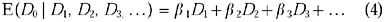where D0 is the deviation from the mean of an individual, D1 the mid-parental deviation (the average deviation of the two parents), D2 the mid-grandparental deviation, and so on. Write this equation as: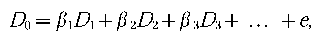where e is a random error term uncorrelated with any of the variables; multiplying this equation by Di and taking expected values, we find that: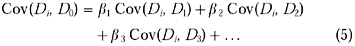Under random mating, Cov(Di, Dj)=rkV/2 m, where k=|ij|, m=min(i, j), and V is the variance of a single observation. Hence we obtain the following set of equations for determining the βis from the ris: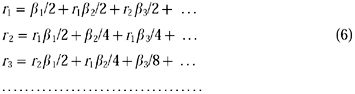These results were obtained by Pearson (1896), 1898) by a slightly different method. Solving these equations with the correlations given in eqn (3) it is not difficult to show that: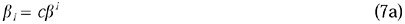where: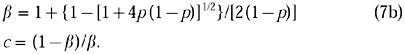The regression coefficients βi sum to unity, which is guaranteed by the value of c. Some numerical values are shown in Table 2. The parameter p, the proportion of patent elements, can be estimated from 2r1, which is the regression of offspring on mid-parent. The ancestral law in eqn (16) is obtained as a special case when p=0.6, as Pearson (1898) observed. It is ironic that Galton's first estimate of the regression of offspring on mid-parent was 3/5 but that the value of 2/3 ‘was afterwards substituted, because the data seemed to admit of that interpretation also, in which case the fraction of two-thirds was preferable as being the more simple expression’ (Galton, 1889, p. 98).

It was shown above that Galton's model (with parameter p) is equivalent to the standard quantitative genetics model under Mendelian inheritance (with heritability h2). Under Galton's model one expects p to be the same for all characters because it is a property of the genetic system, but under the Mendelian model the heritability will vary from character to character. Apart from this, the statistical consequences of the two models are the same.

Under Galton's model of inheritance, we may interpret the contribution of ancestors i generations ago as p(1−p)i−1, the probability that an element patent in the offspring was last patent in an ancestor i generations ago. This is not the same, as Galton plausibly, but wrongly, assumed, as the corresponding coefficient in the multiple regression equation.

This is a logical reconstruction of the relationship between Galton's theory of latent elements and his ancestral law. Galton could not have derived this relationship because the techniques of multiple regression were unknown to him. Pearson developed these techniques but did not use them to incorporate the theory of latent elements, perhaps because he was suspicious of biological theory; he regarded science as the search for statistical regularities, and he disdained speculation about entities underlying them, like gemmules, or latent elements, or genes. Pearson's interpretation of the ancestral law has been discussed recently by Magnello (1998).

## Galton's derivations of the ancestral law

Galton formulated the ancestral law in 1885, and he derived the law by a plausible, though faulty, mathematical argument in an appendix to that paper, which was repeated in almost identical terms in Natural Inheritance (Galton, 1889). He returned to the subject in 1897 with two new arguments, which are even less convincing than the first one (Galton, 1897). These arguments will now be considered in turn.

### Derivation of the law in 1885

As a first step in framing the ancestral law, Galton tried to determine what could be inferred about the deviates of more remote ancestors given the deviate of the mid-parent. To do this, he used his bivariate frequency distribution of the heights of offspring and mid-parents to plot the average mid-parental height against the height of the offspring; the idea was that this would give the same regression as that of mid-grandparent on mid-parent. He found a straight line with a slope of 1/3, so that ‘the most probable mid-parentage of a man is one that deviates only one-third as much as the man does’. In modern terminology: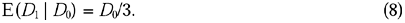Galton used the properties of the bivariate normal distribution to understand the relation between the regressions in eqns (1) and (8). Today the following argument should apply. Under random mating, which held approximately for Galton's data, it is expected that Var(D1)=Var(D0)/2, because D1 is the mean of two randomly chosen heights; and this was empirically true. It is a standard result from regression theory that the slope of the regression of D0 on D1 is Cov(D0, D1)/Var(D1), whereas that of D1 on D0 is Cov(D0, D1)/Var(D0), so that the first slope should be twice the second, as observed.

Galton derived the ancestral law in an appendix to his paper, which is reprinted in the Appendix to this paper and which can be rephrased as follows. The parental, grandparental and more distant ancestral deviations may all affect the offspring deviation because of reversion caused by latent elements; this can be expressed in the multiple regression formula (eqn 4). The regression coefficient β1 reflects the direct effect of the mid-parent on the offspring, β2 reflects the direct effect of the mid-grandparent, and so on.

The regression of offspring on mid-parent is E(D0|D1)=β*D1, say. It is expected that β*>β1 because β* will be influenc ed not only by the direct effect of the mid-parent but also by the indirect effects of more remote ancestors; above-average parents are themselves likely to have above-average parents (grandparents of the offspring), and so on. From eqn (4) can be written: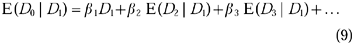Galton had found empirically for human stature that the regression of mid-parent on offspring is 1/3 (eqn 8). This must be the same as that of mid-grandparent on mid-parent, so that: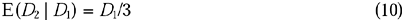and he assumed by analogy that: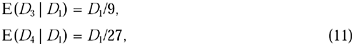and so on. Hence: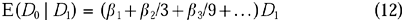so that: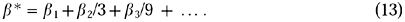To find a relationship between the total regression coefficient β* and the partial regression coefficients βi, Galton considered two limiting hypotheses. Under the constant hypothesis, βi=β for all i, so that: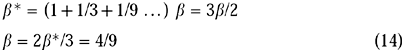because he had found empirically that β*=2/3 for human stature. Under the geometric decrease hypothesis, βii, so that: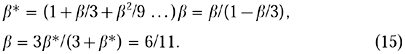Galton now remarks that the two estimates of β are nearly the same, and that their average is nearly 1/2, and he concludes that β1=1/2, β2=1/4, β3=1/8, and so on. This leads to his final result for the multiple regression, the law of ancestral inheritance: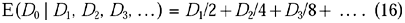Unfortunately, there are several problems in the derivation of this law. First, the coefficients in eqn (11) should be 1/6, 1/12, and so on, rather than 1/9, 1/27, and so on. Secondly, the two results β=4/9 and β=6/11 are obtained under different models, so that there is little logic in averaging them to obtain a value of 1/2; furthermore, Galton abandons the constant hypothesis in favour of the geometric decrease hypothesis as soon as he has obtained the average value of 1/2. Thirdly, neither the constant hypothesis nor the geometric decrease hypothesis is generally true under Galton's model of inheritance; the appropriate hypothesis is βi=cβi (eqn 7). If Galton's argument is reworked under the latter hypothesis, with the coefficients in eqn (11) corrected to 1/6, 1/12, and so on, and with the assumption that the coefficients in the multiple regression formula sum to unity so that c=(1−β)/β, it leads to the result obtained from eqn (7) with p=2/3: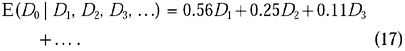It should also be noted that these coefficients do not, as Galton assumed, reflect the contributions of the different ancestors, which are 2/3 for the two parents, 2/9 for the four grandparents, 2/27 for the eight great-grandparents, and so on. Hence the assumption that they sum to unity needs separate justification.

Galton was a pioneer with a very powerful intuition, but he lacked the mathematical skill to develop the technique of multiple regression to its logical conclusion. In view of his mathematical limitations, it is remarkable how close he came to the correct answer under the model he had adopted, which was quite plausible until it was displaced by Mendelism.

### Derivation of the law in 1897

Galton returned to the subject with two new arguments in 1897 (Galton, 1897). He still did not distinguish between the use of the law as a prediction formula and as a representation of ancestral contributions. He presented data verifying the validity of the law as a prediction formula (see Table 1), but his main argument for the law regarded it as representing ancestral contributions:

‘A wide though limited range of observations assures us that the occupier of each ancestral place may contribute something of his own peculiarity, apart from all others, to the heritage of the offspring... Further, it is reasonable to believe that the contributions of parents to children are in the same proportion as those of the grandparents to the parents, of the great-grandparents to the grandparents, and so on; in short, that their total amount is to be expressed by the sum of the terms in an infinite geometric series diminishing to zero. Lastly, it is an essential condition that their total amount should be equal to 1, in order to account for the whole of the heritage. All these conditions are fulfilled by the series of 1/2+(1/2)2+(1/2)3+&c., and by no other.’

In other words, he argues that it is plausible to assume the geometric relationship βii, and that the terms must sum to unity, Σβi=1. Hence β=1/2, giving the ancestral law in eqn (16). This is a different justification of the law from that given in 1885. Galton has abandoned the use of the empirically determined regression of offspring on mid-parent in its derivation and has, instead, adopted a completely a priori approach. In fact, the contribution of the ith ancestral generation under Galton's model is p(1−p)i−1, a modified geometric series with a free parameter to be empirically estimated.

To this argument Galton added another of even more dubious logic:

‘It should be noted that nothing in this statistical law contradicts the generally accepted view that the chief, if not the sole, line of descent runs from germ to germ and not from person to person. The person may be accepted on the whole as a fair representative of the germ, and, being so, the statistical laws which apply to the persons would apply to the germs also, though with less precision in individual cases. Now this law is strictly consonant with the observed binary subdivisions of the germ cells, and the concomitant extrusion and loss of one-half of the several contributions from each of the two parents to the germ-cell of the offspring. The apparent artificiality of the law ceases on these grounds to afford cause for doubt; its close agreement with physiological phenomena ought to give a prejudice in favour of its truth rather than the contrary.’

He is appealing to recent discoveries about the reduction division of the germ cells. He seems to be arguing as follows: (i) parents transmit half of their germ-plasm to their offspring, grandparents one-quarter to their grandchildren, and so on; (ii) therefore an individual receives one-half of his germ-plasm from his parents, one-quarter from his grandparents, and so on; (iii) therefore the same law applies to the inheritance of personal characteristics because the same statistical laws apply to phenotypic and genotypic values. If this is his argument, it is a bad one. The first statement is true, but the second does not follow from it, and the premise of the third statement is false. It is not clear how seriously he intended this argument to be taken.

Thus Galton had come to believe in 1897 that the ancestral law was a logical necessity which could be derived by a priori arguments, although it required empirical verification. In the introduction to this paper he wrote: ‘I stated [the law] briefly and with hesitation in my book ‘Natural Inheritance’, because it was then unsupported by sufficient evidence. Its existence was originally suggested by general considerations, and it might, as will be shown, have been inferred from them with considerable assurance’ (Galton, 1897). After presenting the above two arguments, he concluded: ‘These and the foregoing considerations were referred to when saying that the law might be inferred with considerable assurance a priori’.